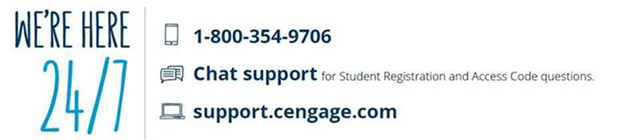Search

AMS 261, Applied Calculus III

Catalog Description: Vector algebra and analytic geometry in 2- and 3-dimensions: multivariable differential calculus and tangent planes; multivariable integral calculus; optimization and Lagrange multipliers; vector calculus including Green's and Stoke's theorems. May not be taken for credit in addition to MAT 203 or 205.

PrerequisitesAMS 161 or MAT 127 or 132 or 142 or MPE level 9.

SBC: STEM+

4 credits: 3 hours of lecture and 1 hour of recitation

SPRING 2019:

The AMS Department recommends the Cengage Unlimited option ( cengage.com/unlimited ) for students who may enroll in future courses where departments use Cengage Publishing textbooks/eBooks

1.Purchase options

Students may purchase direct from the University bookstore or directly from the publisher, Cengage, via WebAssign
Note:  Students should purchase materials using your Stony Brook email to avoid access issues

Choose one of the following items:
a. Cengage Unlimited \$119.99 (a digital subscription service (think Netflix or Apple Music)
b. WebAssign multi-term code \$125 (full sequence WebAssign calculus, with e-book)
c. WebAssign single term \$100 (one semester WebAssign calculus, with e-book))

Cengage Unlimited Subscription includes:

• WebAssign and e-book access for calculus sequence with a free print rental. PLUS ANY Cengage materials you are using across ALL of your courses AND a library of 20,000 e-books.
• Help yourself get a better grade in other courses with our study guides even if you are not using Cengage.
• Access to Quizlet, a hugely popular mobile and web-based study application that helps students make simple learning tools like flashcards and games.
• Access to Career Center tools, students can build employability skills through career readiness tutorials and create a résumé / portfolio with Pathbrite.

2. How to access your course materials after purchase

3. Technical support************************************************************

AMS 261 IS ALSO OFFERED DURING SUMMER SCHOOL. CHECK THE SUMMER SCHOOL BULLETIN FOR TIMES.

Topics
1. Vector algebra and analytic geometry in two and three dimensions  - 6 hours
2. Multivariate Differential Calculus- partial derivatives and gradients, tangent planes - 6 hours
3. Multivariate Integral Calculus:  double and triple integrals, change of variables and Jacobians, polar coordinates, applications to probability - 10 hours
4. Optimization: maxima and minima, Lagrange multipliers - 6 hours
5 . Vector Calculus: vector-valued functions, curves in space, linear integrals, surface integrals, Green's Theorem, Stoke's Theorem - 10 hours
6. Review and Tests - 4 hours

Learning Outcomes for AMS  261, Applied Calculus III

1.) Demonstrate a firm understanding of the vector algebra and the geometry of two-and three-dimensional space. Specifically students should be able to:
* explain and apply both the geometric and algebraic properties of vectors in two and three dimensions.
* compute dot and cross products, and explain their geometric meaning.
* sketch and interpret vector-valued functions in two and three dimensions.
* differentiate and integrate vector-valued functions.
* explain and apply polar, cylindrical and spherical coordinate systems.

2.) Demonstrate an understanding of scalar functions in several dimensions, and the application of differential and integral calculus to multi-variable functions. Specifically students should be able to:
* describe and sketch curves and surfaces in three-dimensional space.
* compute the partial derivatives of multi-variable functions.
* compute and explain directional derivatives and gradients.
* determine the extreme values of multiple variable functions.
* use Lagrange multipliers to solve constrained optimizations problems.
* solve double- and triple-integrals using iterated integration.
* set up double- and triple-integrations problems in both Cartesian and curvilinear coordinate systems.
* explain and apply the use of Jacobians in solving double- and triple-integrals by coordinate substitution.

3.) Demonstrate a understanding of the fundamental concepts of vector algebra and vector calculus; specifically students should be able to:
* describe and sketch vector fields in two and three dimensions.
* compute and interpret line and surface integrals through scalar or vector fields.
* explain and apply Green’s Theorem.
* explain and apply the Divergence Theorem.
* explain and apply Stokes’ Theorem.

4.) Strengthen ability in communicating and translating of mathematical concepts, models to real world settings:
* present solutions to problems in a clear, well-laid out fashion;
* explain key concepts from the class in written English;
*convert problems described in written English into an appropriate mathematical form;
* convert the mathematical solutions into a written answer.
* use the maple computer program as an aid in solving and visualizing mathematical problems.